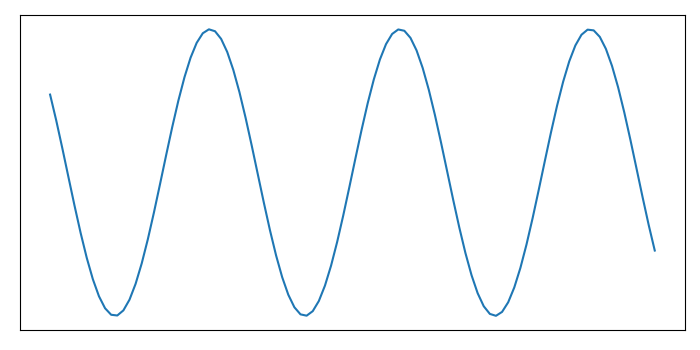# How to turn off the ticks and marks of a matlibplot axes?

To turn off the ticks and marks of a matplotlib axes, we can take the following steps −

• Set the figure size and adjust the padding between and around the subplots.
• Create x and y data points using numpy.
• Plot x and y data points using plot() method.
• Get the current axis of the plot.
• Use set_tick_params() to hide X and Y axes label marks.
• Use set_xticks() and set_yticks() to hide X and Y axes tick marks.
• To display the figure, use show() method.

## Example

import numpy as np
from matplotlib import pyplot as plt

# Set the figure size
plt.rcParams["figure.figsize"] = [7.00, 3.50]
plt.rcParams["figure.autolayout"] = True

# Create x and y data points
x = np.linspace(-10, 10, 100)
y = np.sin(x)

plt.plot(x, y)
ax = plt.gca()

# Hide X and Y axes label marks
ax.xaxis.set_tick_params(labelbottom=False)
ax.yaxis.set_tick_params(labelleft=False)

# Hide X and Y axes tick marks
ax.set_xticks([])
ax.set_yticks([])

plt.show()

## Output

It will produce the following output